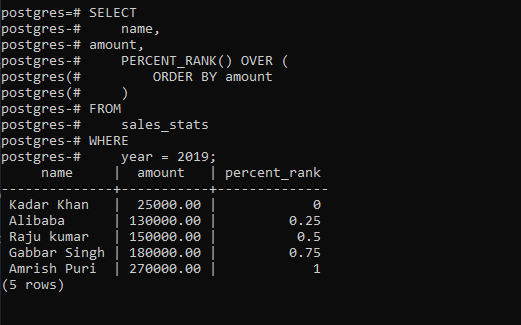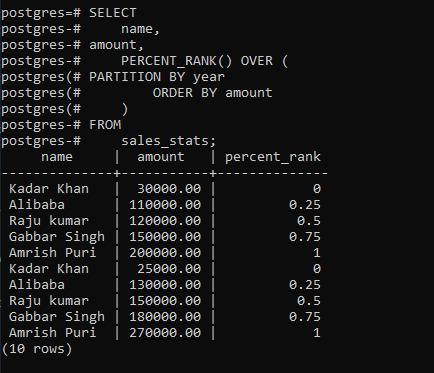# PostgreSQL – PERCENT_RANK Function

• Last Updated : 10 Feb, 2021

In PostgreSQL, the PERCENT_RANK() function is used to evaluate the relative ranking of a value within a given set of values.

The syntax of the PERCENT_RANK() function:

```Syntax:
PERCENT_RANK() OVER (
[PARTITION BY partition_expression, ... ]
ORDER BY sort_expression [ASC | DESC], ...
)
```

Let’s analyze the above syntax:

• The PARTITION BY is an optional clause that is used with the PERCENT_RANK() function to divide rows into multiple partitions. It defaults to a single set.
• The ORDER BY clause is used to set the order in which the resultant query is returned.
• The PERCENT_RANK() function always returns value that is greater than 0 and less than or equal to 1.

Example 1:

First, create two tables named products and product_groups:

```CREATE TABLE product_groups (
group_id serial PRIMARY KEY,
group_name VARCHAR (255) NOT NULL
);

CREATE TABLE products (
product_id serial PRIMARY KEY,
product_name VARCHAR (255) NOT NULL,
price DECIMAL (11, 2),
group_id INT NOT NULL,
FOREIGN KEY (group_id) REFERENCES product_groups (group_id)
);

```

Now add some data to it:

```INSERT INTO product_groups (group_name)
VALUES
('Smartphone'),
('Laptop'),
('Tablet');

INSERT INTO products (product_name, group_id, price)
VALUES
('Microsoft Lumia', 1, 200),
('HTC One', 1, 400),
('Nexus', 1, 500),
('iPhone', 1, 900),
('HP Elite', 2, 1200),
('Lenovo Thinkpad', 2, 700),
('Sony VAIO', 2, 700),
('Dell Vostro', 2, 800),
('Kindle Fire', 3, 150),
('Samsung Galaxy Tab', 3, 200);
```

The following statement uses the PERCENT_RANK() function to calculate the sales percentile of each employee in 2019:

```SELECT
name,
amount,
PERCENT_RANK() OVER (
ORDER BY amount
)
FROM
sales_stats
WHERE
year = 2019;
```

Output:Example 2:

The below statement uses the PERCENT_RANK() function to calculate the sales amount percentile by sales employees in both 2018 and 2019:

```SELECT
name,
amount,
PERCENT_RANK() OVER (
PARTITION BY year
ORDER BY amount
)
FROM
sales_stats;
```

Output:My Personal Notes arrow_drop_up